Newton’s Laws of Motion

# Newton’s Third Law

### Learning Objectives

By the end of the section, you will be able to:

• State Newton’s third law of motion
• Identify the action and reaction forces in different situations
• Apply Newton’s third law to define systems and solve problems of motion

We have thus far considered force as a push or a pull; however, if you think about it, you realize that no push or pull ever occurs by itself. When you push on a wall, the wall pushes back on you. This brings us to Newton’s third law.

Newton’s Third Law of Motion

Whenever one body exerts a force on a second body, the first body experiences a force that is equal in magnitude and opposite in direction to the force that it exerts. Mathematically, if a body A exerts a force $\stackrel{\to }{F}$ on body B, then B simultaneously exerts a force $\text{−}\stackrel{\to }{F}$ on A, or in vector equation form,

${\stackrel{\to }{F}}_{\text{AB}}=\text{−}{\stackrel{\to }{F}}_{\text{BA}}.$

Newton’s third law represents a certain symmetry in nature: Forces always occur in pairs, and one body cannot exert a force on another without experiencing a force itself. We sometimes refer to this law loosely as “action-reaction,” where the force exerted is the action and the force experienced as a consequence is the reaction. Newton’s third law has practical uses in analyzing the origin of forces and understanding which forces are external to a system.

We can readily see Newton’s third law at work by taking a look at how people move about. Consider a swimmer pushing off the side of a pool ((Figure)). She pushes against the wall of the pool with her feet and accelerates in the direction opposite that of her push. The wall has exerted an equal and opposite force on the swimmer. You might think that two equal and opposite forces would cancel, but they do not because they act on different systems. In this case, there are two systems that we could investigate: the swimmer and the wall. If we select the swimmer to be the system of interest, as in the figure, then ${F}_{\text{wall on feet}}$ is an external force on this system and affects its motion. The swimmer moves in the direction of this force. In contrast, the force ${F}_{\text{feet on wall}}$ acts on the wall, not on our system of interest. Thus, ${F}_{\text{feet on wall}}$ does not directly affect the motion of the system and does not cancel ${F}_{\text{wall on feet}}.$ The swimmer pushes in the direction opposite that in which she wishes to move. The reaction to her push is thus in the desired direction. In a free-body diagram, such as the one shown in (Figure), we never include both forces of an action-reaction pair; in this case, we only use ${F}_{\text{wall on feet}}$, not ${F}_{\text{feet on wall}}$.

When the swimmer exerts a force on the wall, she accelerates in the opposite direction; in other words, the net external force on her is in the direction opposite of ${F}_{\text{feet on wall}}.$ This opposition occurs because, in accordance with Newton’s third law, the wall exerts a force ${F}_{\text{wall on feet}}$ on the swimmer that is equal in magnitude but in the direction opposite to the one she exerts on it. The line around the swimmer indicates the system of interest. Thus, the free-body diagram shows only ${F}_{\text{wall on feet}},$ w (the gravitational force), and BF, which is the buoyant force of the water supporting the swimmer’s weight. The vertical forces w and BF cancel because there is no vertical acceleration.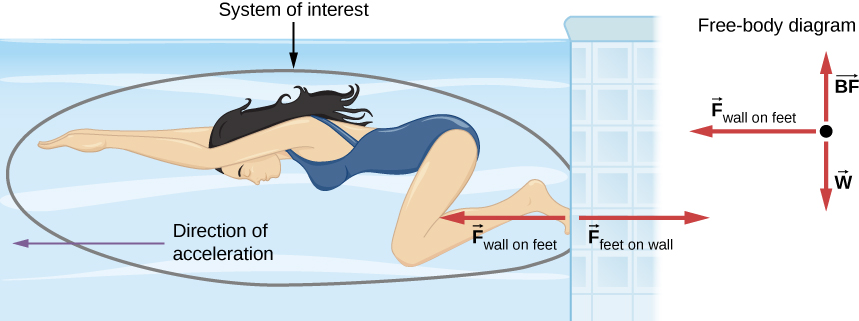Other examples of Newton’s third law are easy to find:

• As a professor paces in front of a whiteboard, he exerts a force backward on the floor. The floor exerts a reaction force forward on the professor that causes him to accelerate forward.
• A car accelerates forward because the ground pushes forward on the drive wheels, in reaction to the drive wheels pushing backward on the ground. You can see evidence of the wheels pushing backward when tires spin on a gravel road and throw the rocks backward.
• Rockets move forward by expelling gas backward at high velocity. This means the rocket exerts a large backward force on the gas in the rocket combustion chamber; therefore, the gas exerts a large reaction force forward on the rocket. This reaction force, which pushes a body forward in response to a backward force, is called thrust. It is a common misconception that rockets propel themselves by pushing on the ground or on the air behind them. They actually work better in a vacuum, where they can more readily expel the exhaust gases.
• Helicopters create lift by pushing air down, thereby experiencing an upward reaction force.
• Birds and airplanes also fly by exerting force on the air in a direction opposite that of whatever force they need. For example, the wings of a bird force air downward and backward to get lift and move forward.
• An octopus propels itself in the water by ejecting water through a funnel from its body, similar to a jet ski.
• When a person pulls down on a vertical rope, the rope pulls up on the person ((Figure)).
When the mountain climber pulls down on the rope, the rope pulls up on the mountain climber.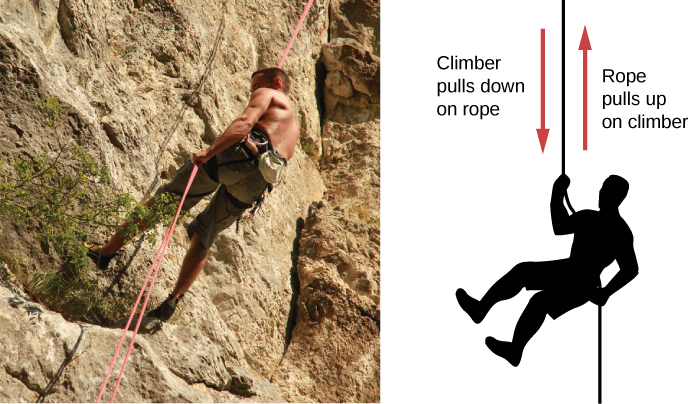There are two important features of Newton’s third law. First, the forces exerted (the action and reaction) are always equal in magnitude but opposite in direction. Second, these forces are acting on different bodies or systems: A’s force acts on B and B’s force acts on A. In other words, the two forces are distinct forces that do not act on the same body. Thus, they do not cancel each other.

For the situation shown in (Figure), the third law indicates that because the chair is pushing upward on the boy with force $\stackrel{\to }{C},$ he is pushing downward on the chair with force $\text{−}\stackrel{\to }{C}.$ Similarly, he is pushing downward with forces $\text{−}\stackrel{\to }{F}$ and $\text{−}\stackrel{\to }{T}$ on the floor and table, respectively. Finally, since Earth pulls downward on the boy with force $\stackrel{\to }{w},$ he pulls upward on Earth with force $\text{−}\stackrel{\to }{w}$. If that student were to angrily pound the table in frustration, he would quickly learn the painful lesson (avoidable by studying Newton’s laws) that the table hits back just as hard.

A person who is walking or running applies Newton’s third law instinctively. For example, the runner in (Figure) pushes backward on the ground so that it pushes him forward.

The runner experiences Newton’s third law. (a) A force is exerted by the runner on the ground. (b) The reaction force of the ground on the runner pushes him forward.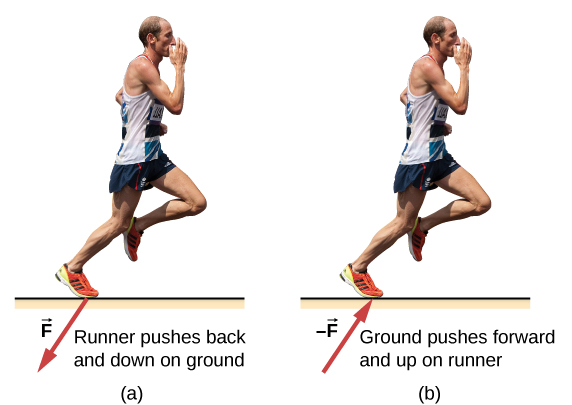Forces on a Stationary Object
The package in (Figure) is sitting on a scale. The forces on the package are $\stackrel{\to }{S},$ which is due to the scale, and $\text{−}\stackrel{\to }{w},$ which is due to Earth’s gravitational field. The reaction forces that the package exerts are $\text{−}\stackrel{\to }{S}$ on the scale and $\stackrel{\to }{w}$ on Earth. Because the package is not accelerating, application of the second law yields

$\stackrel{\to }{S}-\stackrel{\to }{w}=m\stackrel{\to }{a}=\stackrel{\to }{0},$

so

$\stackrel{\to }{S}=\stackrel{\to }{w}.$

Thus, the scale reading gives the magnitude of the package’s weight. However, the scale does not measure the weight of the package; it measures the force $\text{−}\stackrel{\to }{S}$ on its surface. If the system is accelerating, $\stackrel{\to }{S}$ and $\text{−}\stackrel{\to }{w}$ would not be equal, as explained in Applications of Newton’s Laws.

(a) The forces on a package sitting on a scale, along with their reaction forces. The force $\stackrel{\to }{w}$ is the weight of the package (the force due to Earth’s gravity) and $\stackrel{\to }{S}$ is the force of the scale on the package. (b) Isolation of the package-scale system and the package-Earth system makes the action and reaction pairs clear.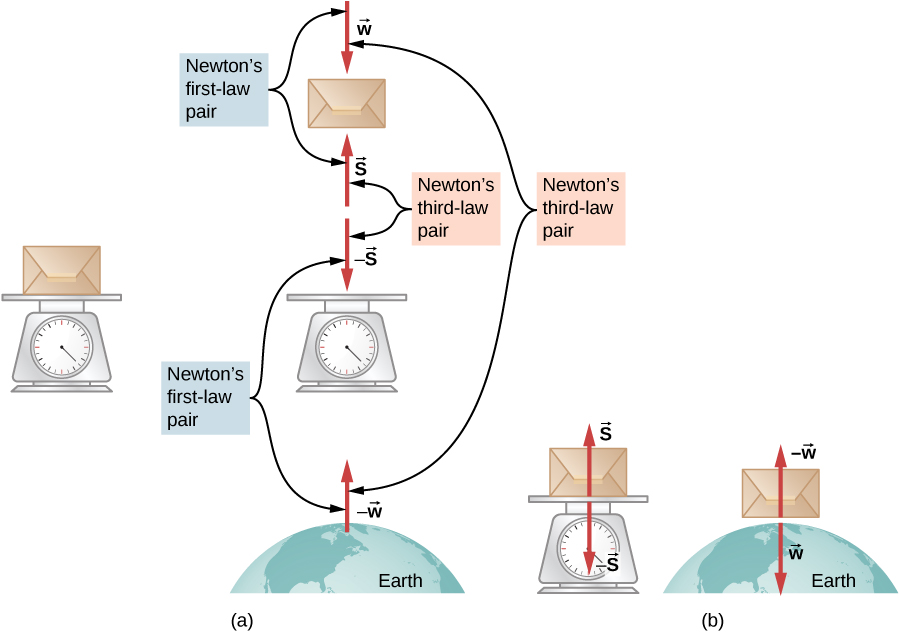Getting Up to Speed: Choosing the Correct System
A physics professor pushes a cart of demonstration equipment to a lecture hall ((Figure)). Her mass is 65.0 kg, the cart’s mass is 12.0 kg, and the equipment’s mass is 7.0 kg. Calculate the acceleration produced when the professor exerts a backward force of 150 N on the floor. All forces opposing the motion, such as friction on the cart’s wheels and air resistance, total 24.0 N.

A professor pushes the cart with her demonstration equipment. The lengths of the arrows are proportional to the magnitudes of the forces (except for $\stackrel{\to }{f}\text{,}$ because it is too small to drawn to scale). System 1 is appropriate for this example, because it asks for the acceleration of the entire group of objects. Only ${\stackrel{\to }{F}}_{\text{floor}}$ and $\stackrel{\to }{f}$ are external forces acting on System 1 along the line of motion. All other forces either cancel or act on the outside world. System 2 is chosen for the next example so that ${\stackrel{\to }{F}}_{\text{prof}}$ is an external force and enters into Newton’s second law. The free-body diagrams, which serve as the basis for Newton’s second law, vary with the system chosen.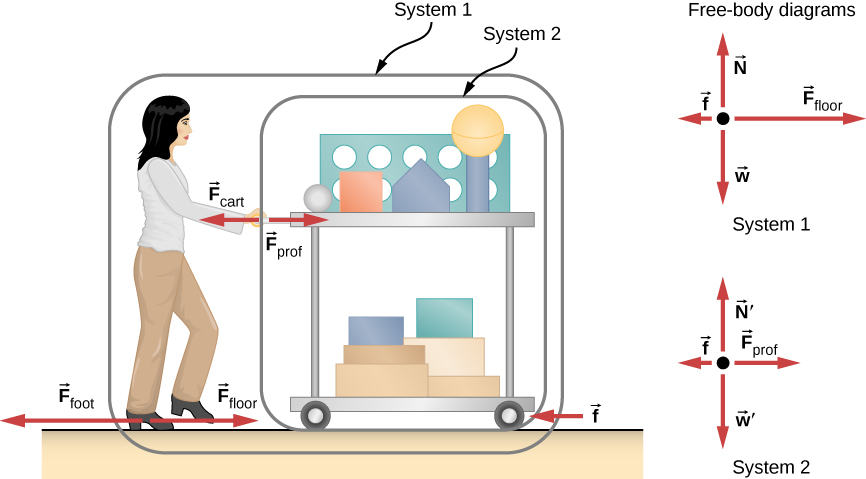Strategy
Since they accelerate as a unit, we define the system to be the professor, cart, and equipment. This is System 1 in (Figure). The professor pushes backward with a force ${F}_{\text{foot}}$ of 150 N. According to Newton’s third law, the floor exerts a forward reaction force ${F}_{\text{floor}}$ of 150 N on System 1. Because all motion is horizontal, we can assume there is no net force in the vertical direction. Therefore, the problem is one-dimensional along the horizontal direction. As noted, friction f opposes the motion and is thus in the opposite direction of ${F}_{\text{floor}}.$ We do not include the forces ${F}_{\text{prof}}$ or ${F}_{\text{cart}}$ because these are internal forces, and we do not include ${F}_{\text{foot}}$ because it acts on the floor, not on the system. There are no other significant forces acting on System 1. If the net external force can be found from all this information, we can use Newton’s second law to find the acceleration as requested. See the free-body diagram in the figure.

Solution
Newton’s second law is given by

$a=\frac{{F}_{\text{net}}}{m}.$

The net external force on System 1 is deduced from (Figure) and the preceding discussion to be

${F}_{\text{net}}={F}_{\text{floor}}-f=150\phantom{\rule{0.2em}{0ex}}\text{N}-24.0\phantom{\rule{0.2em}{0ex}}\text{N}=126\phantom{\rule{0.2em}{0ex}}\text{N}\text{.}$

The mass of System 1 is

$m=\left(65.0+12.0+7.0\right)\phantom{\rule{0.2em}{0ex}}\text{kg}=84\phantom{\rule{0.2em}{0ex}}\text{kg}\text{.}$

These values of ${F}_{\text{net}}$ and m produce an acceleration of

$a=\frac{{F}_{\text{net}}}{m}=\frac{126\phantom{\rule{0.2em}{0ex}}\text{N}}{84\phantom{\rule{0.2em}{0ex}}\text{kg}}=1.5\phantom{\rule{0.2em}{0ex}}{\text{m/s}}^{2}.$

Significance
None of the forces between components of System 1, such as between the professor’s hands and the cart, contribute to the net external force because they are internal to System 1. Another way to look at this is that forces between components of a system cancel because they are equal in magnitude and opposite in direction. For example, the force exerted by the professor on the cart results in an equal and opposite force back on the professor. In this case, both forces act on the same system and therefore cancel. Thus, internal forces (between components of a system) cancel. Choosing System 1 was crucial to solving this problem.

Force on the Cart: Choosing a New System
Calculate the force the professor exerts on the cart in (Figure), using data from the previous example if needed.

Strategy
If we define the system of interest as the cart plus the equipment (System 2 in (Figure)), then the net external force on System 2 is the force the professor exerts on the cart minus friction. The force she exerts on the cart, ${F}_{\text{prof}}$, is an external force acting on System 2. ${F}_{\text{prof}}$ was internal to System 1, but it is external to System 2 and thus enters Newton’s second law for this system.

Solution
Newton’s second law can be used to find ${F}_{\text{prof}}.$ We start with

$a=\frac{{F}_{\text{net}}}{m}.$

The magnitude of the net external force on System 2 is

${F}_{\text{net}}={F}_{\text{prof}}-f.$

We solve for ${F}_{\text{prof}}$, the desired quantity:

${F}_{\text{prof}}={F}_{\text{net}}+f.$

The value of f is given, so we must calculate net ${F}_{\text{net}}.$ That can be done because both the acceleration and the mass of System 2 are known. Using Newton’s second law, we see that

${F}_{\text{net}}=ma,$

where the mass of System 2 is 19.0 kg ($m=12.0\phantom{\rule{0.2em}{0ex}}\text{kg}+7.0\phantom{\rule{0.2em}{0ex}}\text{kg}$) and its acceleration was found to be $a=1.5\phantom{\rule{0.2em}{0ex}}{\text{m/s}}^{2}$ in the previous example. Thus,

${F}_{\text{net}}=ma=\left(19.0\phantom{\rule{0.2em}{0ex}}\text{kg}\right)\left(1.5\phantom{\rule{0.2em}{0ex}}{\text{m/s}}^{2}\right)=29\phantom{\rule{0.2em}{0ex}}\text{N}\text{.}$

Now we can find the desired force:

${F}_{\text{prof}}={F}_{\text{net}}+f=29\phantom{\rule{0.2em}{0ex}}\text{N}+24.0\phantom{\rule{0.2em}{0ex}}\text{N}=53\phantom{\rule{0.2em}{0ex}}\text{N}\text{.}$

Significance
This force is significantly less than the 150-N force the professor exerted backward on the floor. Not all of that 150-N force is transmitted to the cart; some of it accelerates the professor. The choice of a system is an important analytical step both in solving problems and in thoroughly understanding the physics of the situation (which are not necessarily the same things).

Check Your Understanding Two blocks are at rest and in contact on a frictionless surface as shown below, with ${m}_{1}=2.0\phantom{\rule{0.2em}{0ex}}\text{kg},$ ${m}_{2}=6.0\phantom{\rule{0.2em}{0ex}}\text{kg},$ and applied force 24 N. (a) Find the acceleration of the system of blocks. (b) Suppose that the blocks are later separated. What force will give the second block, with the mass of 6.0 kg, the same acceleration as the system of blocks?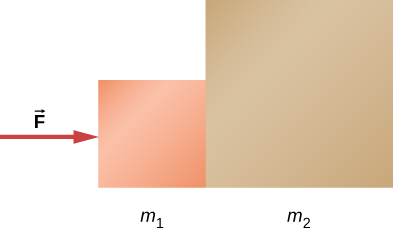a. $3.0\phantom{\rule{0.2em}{0ex}}\text{m}\text{/}{\text{s}}^{2}$; b. 18 N

View this video to watch examples of action and reaction.

View this video to watch examples of Newton’s laws and internal and external forces.

### Summary

• Newton’s third law of motion represents a basic symmetry in nature, with an experienced force equal in magnitude and opposite in direction to an exerted force.
• Two equal and opposite forces do not cancel because they act on different systems.
• Action-reaction pairs include a swimmer pushing off a wall, helicopters creating lift by pushing air down, and an octopus propelling itself forward by ejecting water from its body. Rockets, airplanes, and cars are pushed forward by a thrust reaction force.
• Choosing a system is an important analytical step in understanding the physics of a problem and solving it.

### Conceptual Questions

Identify the action and reaction forces in the following situations: (a) Earth attracts the Moon, (b) a boy kicks a football, (c) a rocket accelerates upward, (d) a car accelerates forward, (e) a high jumper leaps, and (f) a bullet is shot from a gun.

a. action: Earth pulls on the Moon, reaction: Moon pulls on Earth; b. action: foot applies force to ball, reaction: ball applies force to foot; c. action: rocket pushes on gas, reaction: gas pushes back on rocket; d. action: car tires push backward on road, reaction: road pushes forward on tires; e. action: jumper pushes down on ground, reaction: ground pushes up on jumper; f. action: gun pushes forward on bullet, reaction: bullet pushes backward on gun.

Suppose that you are holding a cup of coffee in your hand. Identify all forces on the cup and the reaction to each force.

(a) Why does an ordinary rifle recoil (kick backward) when fired? (b) The barrel of a recoilless rifle is open at both ends. Describe how Newton’s third law applies when one is fired. (c) Can you safely stand close behind one when it is fired?

a. The rifle (the shell supported by the rifle) exerts a force to expel the bullet; the reaction to this force is the force that the bullet exerts on the rifle (shell) in opposite direction. b. In a recoilless rifle, the shell is not secured in the rifle; hence, as the bullet is pushed to move forward, the shell is pushed to eject from the opposite end of the barrel. c. It is not safe to stand behind a recoilless rifle.

### Problems

(a) What net external force is exerted on a 1100.0-kg artillery shell fired from a battleship if the shell is accelerated at $2.40\phantom{\rule{0.2em}{0ex}}×\phantom{\rule{0.2em}{0ex}}{10}^{4}\phantom{\rule{0.2em}{0ex}}{\text{m/s}}^{2}?$ (b) What is the magnitude of the force exerted on the ship by the artillery shell, and why?

a. ${F}_{\text{net}}=2.64\phantom{\rule{0.2em}{0ex}}×\phantom{\rule{0.2em}{0ex}}{10}^{7}\phantom{\rule{0.2em}{0ex}}\text{N;}$ b. The force exerted on the ship is also $2.64\phantom{\rule{0.2em}{0ex}}×\phantom{\rule{0.2em}{0ex}}{10}^{7}\phantom{\rule{0.2em}{0ex}}\text{N}$ because it is opposite the shell’s direction of motion.

A brave but inadequate rugby player is being pushed backward by an opposing player who is exerting a force of 800.0 N on him. The mass of the losing player plus equipment is 90.0 kg, and he is accelerating backward at $1.20\phantom{\rule{0.2em}{0ex}}{\text{m/s}}^{2}$. (a) What is the force of friction between the losing player’s feet and the grass? (b) What force does the winning player exert on the ground to move forward if his mass plus equipment is 110.0 kg?

A history book is lying on top of a physics book on a desk, as shown below; a free-body diagram is also shown. The history and physics books weigh 14 N and 18 N, respectively. Identify each force on each book with a double subscript notation (for instance, the contact force of the history book pressing against physics book can be described as ${\stackrel{\to }{F}}_{\text{HP}}$), and determine the value of each of these forces, explaining the process used.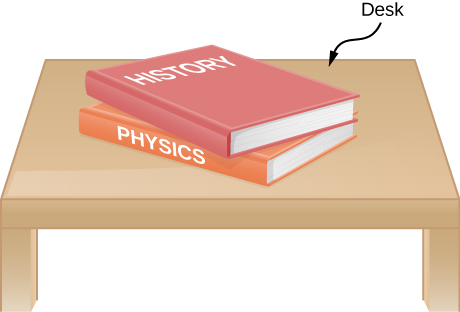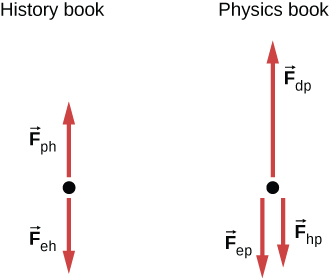Because the weight of the history book is the force exerted by Earth on the history book, we represent it as ${\stackrel{\to }{F}}_{\text{EH}}=-14\stackrel{^}{j}\phantom{\rule{0.2em}{0ex}}\text{N}\text{.}$ Aside from this, the history book interacts only with the physics book. Because the acceleration of the history book is zero, the net force on it is zero by Newton’s second law: ${\stackrel{\to }{F}}_{\text{PH}}+{\stackrel{\to }{F}}_{\text{EH}}=\stackrel{\to }{0},$ where ${\stackrel{\to }{F}}_{\text{PH}}$ is the force exerted by the physics book on the history book. Thus, ${\stackrel{\to }{F}}_{\text{PH}}=\text{−}{\stackrel{\to }{F}}_{\text{EH}}=\text{−}\left(-14\stackrel{^}{j}\right)\phantom{\rule{0.2em}{0ex}}\text{N}=14\stackrel{^}{j}\phantom{\rule{0.2em}{0ex}}\text{N}\text{.}$ We find that the physics book exerts an upward force of magnitude 14 N on the history book. The physics book has three forces exerted on it: ${\stackrel{\to }{F}}_{\text{EP}}$ due to Earth, ${\stackrel{\to }{F}}_{\text{HP}}$ due to the history book, and ${\stackrel{\to }{F}}_{\text{DP}}$ due to the desktop. Since the physics book weighs 18 N, ${\stackrel{\to }{F}}_{\text{EP}}=-18\stackrel{^}{j}\phantom{\rule{0.2em}{0ex}}\text{N}\text{.}$ From Newton’s third law, ${\stackrel{\to }{F}}_{\text{HP}}=\text{−}{\stackrel{\to }{F}}_{\text{PH}},$ so ${\stackrel{\to }{F}}_{\text{HP}}=-14\stackrel{^}{j}\phantom{\rule{0.2em}{0ex}}\text{N}\text{.}$ Newton’s second law applied to the physics book gives $\sum \stackrel{\to }{F}=\stackrel{\to }{0},$ or ${\stackrel{\to }{F}}_{\text{DP}}+{\stackrel{\to }{F}}_{\text{EP}}+{\stackrel{\to }{F}}_{\text{HP}}=\stackrel{\to }{0},$ so ${\stackrel{\to }{F}}_{\text{DP}}=\text{−}\left(-18\stackrel{^}{j}\right)-\left(-14\stackrel{^}{j}\right)=32\stackrel{^}{j}\phantom{\rule{0.2em}{0ex}}\text{N}\text{.}$ The desk exerts an upward force of 32 N on the physics book. To arrive at this solution, we apply Newton’s second law twice and Newton’s third law once.

A truck collides with a car, and during the collision, the net force on each vehicle is essentially the force exerted by the other. Suppose the mass of the car is 550 kg, the mass of the truck is 2200 kg, and the magnitude of the truck’s acceleration is $10\phantom{\rule{0.2em}{0ex}}{\text{m/s}}^{2}$. Find the magnitude of the car’s acceleration.

### Glossary

Newton’s third law of motion
whenever one body exerts a force on a second body, the first body experiences a force that is equal in magnitude and opposite in direction to the force that it exerts
thrust
reaction force that pushes a body forward in response to a backward force